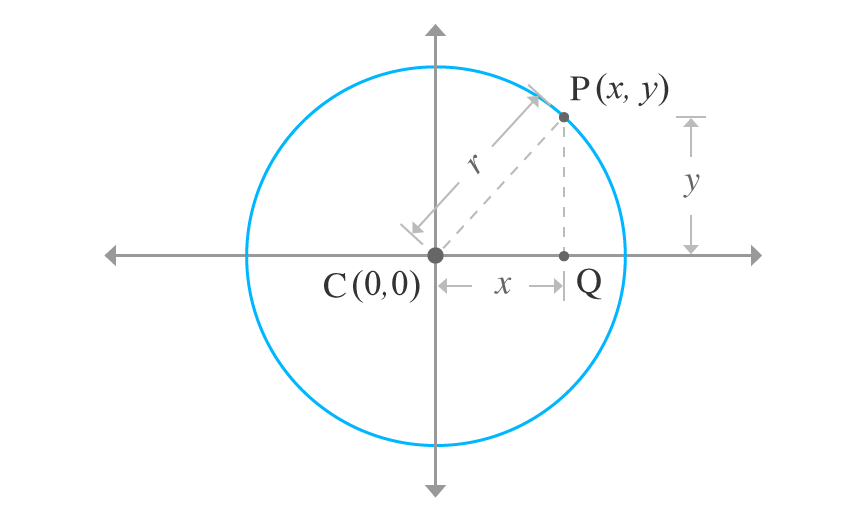# Equation of a circle when Centre coincides with the origin

## Equation

$x^2 + y^2 = r^2$

### Proof

Consider a circle in two dimensional space and centre of the circle coincides with the origin of the two dimensional Cartesian coordinate system. It is assumed that the radius of the circle is $r$. Consider a point $P$ on the circumference of the circle and its coordinates are $x$ and $y$. Therefore, the point is $P (x, y)$.Connect centre of the circle with point $P$ by a line. Draw a line from point $P$ and it should be parallel to $y$-axis and perpendicular to $x$-axis. The drawn line intersects the $x$-axis at point $Q$. The geometric process formed a right angled triangle $PCQ$.

As per right angled triangle $PCQ$

1. Length of the opposite side is $PQ = y$
2. Length of the adjacent side is $CQ = x$
3. Length of the hypotenuse $CP = r$

According to Pythagorean Theorem, the sides of $\Delta PCQ$ is expressed as follows.

${CP}^2 = {CQ}^2 + {PQ}^2$

$\implies r^2 = x^2 + y^2$

$\therefore \,\,\,\,\, x^2 + y^2 = r^2$

It is a circle’s equation in algebraic form when the centre of the circle coincides with the origin of the coordinate system.

Latest Math Topics
Latest Math Problems

A best free mathematics education website for students, teachers and researchers.

###### Maths Topics

Learn each topic of the mathematics easily with understandable proofs and visual animation graphics.

###### Maths Problems

Learn how to solve the maths problems in different methods with understandable steps.

Learn solutions

###### Subscribe us

You can get the latest updates from us by following to our official page of Math Doubts in one of your favourite social media sites.